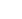# Regression analysis

It is very similar to simple regression except that you have more than one predictor variables in the equation. To look at the relationship between two variables

1. Go to the Analyze menu- Regression-click on Curve Estimation.
• Curve estimation dialogue box opens
1. Transfer the Dependent variable to the Dependent(s) variable box and the Independent variable to the Independent variable box

-the dependent variable is predicted using the independent variable

1. Deselect the Plot modes check box
2. Select the Display ANOVA table check box
3. Under Models, deselect the Linear check box and select the Cubic check box.
4. Click the Ok button

Automated page speed optimizations for fast site performance